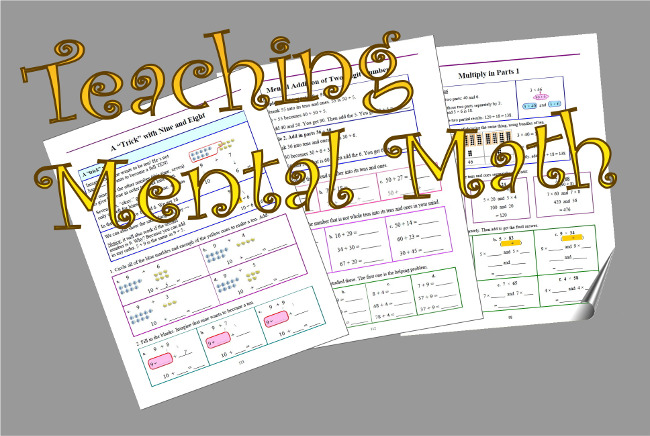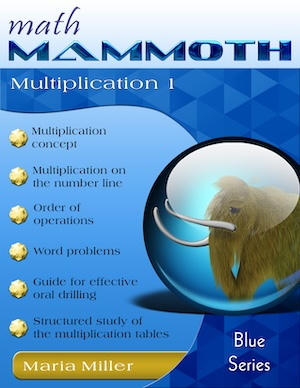A 40% off sale for the large Math Mammoth bundles at Homeschool Buyers Club. The offer expires on 3/31/2023.
^
You are here: HomeLessons → Mental math

# 7 practical tips for mental math (that ANYONE can use!)

You have most likely heard about mental math — the ability to do calculations in one's head — and how important it is for children to learn it. But why is it important? Because mental math relates to NUMBER SENSE: the ability to manipulate numbers in one's head in various ways in order to do calculations. And number sense, in return, has been proven to predict a student's success in algebra. Essentially, what we do with variables in algebra is the same as what students can learn do with numbers in the lower grades.

People with number sense use numbers flexibly. They are able to take them apart and put them together in various ways in order to do calculations. It is quite similar to being able to "PLAY" with words in order to make interesting sentences, or being able to play with chords and melodies in order to make songs.

But mental math/number sense isn't just for "math whizzes" — quite the contrary! EVERYONE can learn the basics of it, and it will make learning math and algebra so much easier! We expect our children to learn lots of English words and to be able to put those words together in many different ways to form sentences, so why not expect them to do the same with numbers? And they can, as long as they are shown the basics and shown examples of how it happens. So let's get on to the practical part of this writing: mental math strategies for EVERYONE.1. The "9-trick".

To add 9 to any number, first add 10, and then subtract 1. In my Math Mammoth books, I give children this storyline where nine really badly wants to be 10… so, it asks this other number for "one". The other number then becomes one less. For example, we change the addition 9 + 7 to 10 + 6, which is much easier to solve.### Math Mammoth Multiplication 1

A self-teaching worktext that covers the concept of multiplication from various angles, word problems, a guide for structural drilling, and a complete study of all 12 multiplication tables.

But this "trick" expands. Can you think of an easy way to add 76 + 99? Change it to 75 + 100. How about 385 + 999?

How would you add 39 + 28 in your head? Let 39 become 40… which reduces 28 to 27. The addition is now 40 + 27. Yet another way is by thinking of compensation: 39 is one less than 40, and 28 is two less than 30. So, their sum is three less than 70.

2. Doubles + 1.

Encourage children to memorize the doubles from 1 + 1 through 9 + 9. After that, a whole lot of other addition facts are at their fingertips: the ones we can term "doubles plus one more". For example, 5 + 6 is just one more than 5 + 5, or 9 + 8 is just one more than 8 + 8.

Once you know that 7 + 8 = 15, then you will also be able to do all these additions in your head:

• 70 + 80 is 15 tens, or 150
• 700 + 800 is 15 hundreds, or 1500
• 27 + 8 is 20 and 15, which is 35. Or, think this way: since 7 + 8 is five more than ten, then 27 + 8 is five more than the next ten.

This is a very important principle, based on the connection between addition and subtraction. Children really don't need to memorize subtraction facts as such, if they can use this principle. For example, to find 8 − 6, think, "Six plus what number makes 8?" In other words, think of the missing number addition 6 + ___ = 8. The answer to that is also the answer to 8 − 6.

This principle comes in especially handy with subtractions such as 13 − 7, 17 − 8, 16 − 9, and other basic subtraction facts where the minuend is between 10 and 20. But you can also use it in multitudes of other situations. For example, 63 − 52 is easier to solve by thinking of addition: 52 + 11 makes 63, so the answer to 63 − 52 is 11.

5. Five times a number.

Turning our attention to multiplication now, here's a nifty trick you might not know about. To find 5 times any number, first multiply that number times ten, then take half of that. For example, 5 × 48 can be found by multiplying 10 × 48 = 480, and taking half of the result, which gives us 240. Of course, you can also use this strategy for such multiplication facts as 5 × 7 or 5 × 9.

6. Four and eight times a number.

If you can double numbers, you already have this down pat! To find four times a number, double that number twice. For example, what is 4 × 59? First find double 59, which is 118. Then double that, and you get 236.

Similarly, eight times a number just means doubling three times. As an example, to find 8 × 35 means doubling 35 to get 70, doubling 70 to get 140, and (once more) doubling 140 to get 280. However, personally I would transform 8 × 35 into 4 × 70 (you double one factor and halve the other), which is easy to solve to be 280.

7. Multiply in parts.

This strategy is very simple, and in fact it is the foundation for the standard multiplication algorithm. You can find 3 × 74 mentally by multiplying 3 × 70 and 3 × 4, and adding the results. We get 210 + 12 = 222. Another example: 6 × 218 is 6 × 200 and 6 × 10 and 6 × 8, which is 1200 + 60 + 48 = 1308.

I hope these little strategies or principles will inspire you not only to teach your children more mental math, but also to use mental math in your everyday life. It's never too late to start playing with numbers!

By Maria Miller

Article originally published in HomeschoolMagazine.com.

WAIT!

Receive my monthly collection of math tips & resources directly in your inbox — and get a FREE Math Mammoth book!You can unsubscribe at any time.

### Math Mammoth Tour

Confused about the different options? Take a virtual email tour around Math Mammoth! You'll receive:

An initial email to download your GIFT of over 400 free worksheets and sample pages from my books. Six other "TOURSTOP" emails that explain the important things and commonly asked questions concerning Math Mammoth curriculum. (Find out the differences between all these different-colored series!)

This way, you'll have time to digest the information over one or two weeks, plus an opportunity to ask me personally about the curriculum.
A monthly collection of math teaching tips & Math Mammoth updates (unsubscribe any time)### "Mini" Math Teaching Course

This is a little "virtual" 2-week course, where you will receive emails on important topics on teaching math, including:

- How to help a student who is behind
- Troubles with word problems
- Teaching multiplication tables
- Why fractions are so difficult
- The value of mistakes
- Should you use timed tests
- And more!

A GIFT of over 400 free worksheets and sample pages from my books right in the very beginning.A monthly collection of math teaching tips & Math Mammoth updates (unsubscribe any time)
Enter your email to receive math teaching tips, resources, Math Mammoth news & sales, humor, and more! I tend to send out these tips about once monthly, near the beginning of the month, but occasionally you may hear from me twice per month (and sometimes less often).• A GIFT of over 400 free worksheets and sample pages from my books.